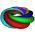## Sunday, October 7, 2012

### Practice Problem

First one to post comment with answer wins!

Go.

Mr. Morrissey

Source: Mu Alpha Theta

#### 2 comments:

1.Surely you should require a complete solution as well ;)

Since I am not satisfied with just providing a standard algebra solution (:D), here is a vector solution:

The line 2x + 7y = 3 can be parametrized as (x, y) = (7t + 3/2, -2t) = (3/2, 0) + (7, -2)t. One sees that the direction vector of this parametrization is (7, -2). The direction vector v := (v1, v2) of any perpendicular line must satisfy v*(7, -2) = 0 (where * is dot product). One such v can be (2, 7). Since we also know that (-5, 0) should be on our desired line, it can be parametrized by (x, y) = (-5, 0) + (2, 7)t = (2t - 5, 7t).

Now it remains to express this line in standard form. This is easy, because for any t, we have that 7(2t - 5) - 2(7t) = -35. Therefore, if (x, y) = (-2t - 5, 7t), then 7x - 2y = -35. Putting this into slope-intercept form yields y = (7/2)x + 35/2.

2.Because I am kind, I will also provide a simpler solution:

The slope of 2x + 7y = 3 is -2/7; so the slope of any perpendicular line will be 7/2. We know that (-5, 0) is on this perpendicular line, so that is enough to reduce the line to the one with equation y = 7/2 (x + 5) = (7/2)x + 35/2.